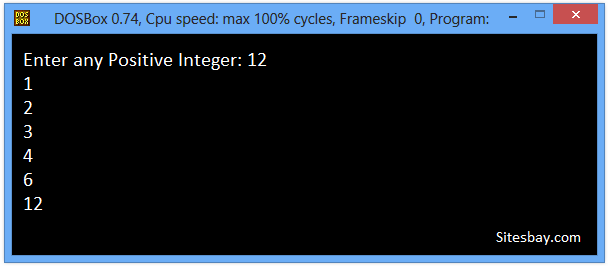# Find All Factors of a Number Program in C++

## C++ Program to Find All Factors of Any Number

Factors of any number are those numbers that are multiplied to get a number. For example: 6 and 3 are factors of 18 as 6*3=18. Similarly other factors of 15 are 1 and 18 as 18*1=18.

To understand below example, you have must knowledge of following C++ programming topics; For Loop in C++ and If Else Statement in C++

## C++ Program to Display Factors of a Number

```#include<iostream.h>
#include<conio.h>

void main()
{
int n, i;
clrscr();
cout<<"Enter any Positive Integer: ";
cin>>n;

cout<<"Factors of "<<n<<" are: "<<endl;
for(i = 1; i <= n; ++i)
{
if(n % i == 0)
cout<<i<<endl;
}
getch();
}

```

In the above program, the for loop runs from 1 to num. The number is divided by i and if the remainder is 0, then i is a factor of num and is printed.

## Output

```Enter any Positive Integer: 12
Factors of 12 are:
1
2
3
4
6
12
```

## Output

```Enter any Positive Integer: 25
Factors of 25 are:
1
5
25
```## Pure VPN Privide Lowest Price VPN Just @ \$1.65. Per Month with Non Detected IP Lowest Price Non Detected IP VPN